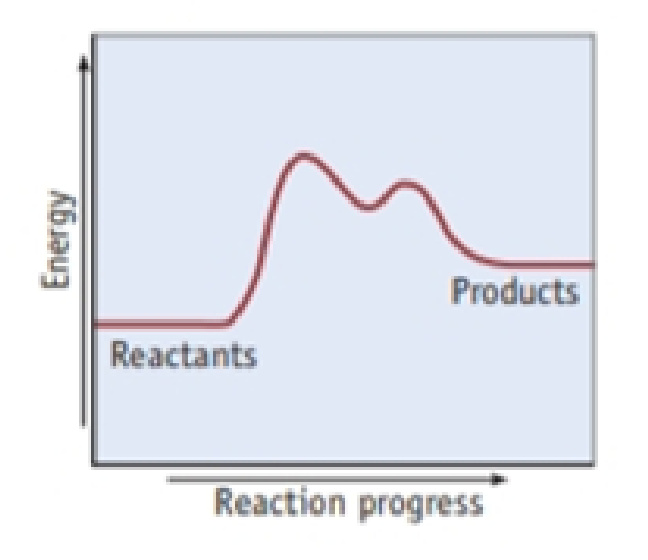Chapter 14, Problem 42PS

Chapter
Section
Textbook Problem

Answer the following questions based on the diagram below. (a) Is the reaction exothermic or endothermic? (b) Does the reaction occur in more than one step? If so, how many?(a)

Interpretation Introduction

Interpretation:

From the given energy profile diagram the type of reaction as either exothermic or endothermic has to be explained.

Concept introduction:

In order to establish the plausibility of a mechanism, one must compare the rate law of the rate determining step to the experimentally determined rate law.

Energy profile diagram: It is diagrammatic representation of the reaction pathway which is depicted along the reaction coordinates that is energy and time as the reactions proceeds in product formation.

Rate determining step: In a chemical reaction the rate determining step is the slowest step in which the rate of the reaction depends on the rate of that slowest step.

Rate law: It is generally the rate equation that consists of the reaction rate with the concentration or the pressures of the reactants and constant parameters.

Activation energy: It is defined as the minimum energy required by the reacting species in order to undergo chemical reaction.

Intermediate species: It is the species formed during the middle of the chemical reaction between the reactant and the desired product.

Exothermic reaction: The reaction is termed as exothermic reaction if energy is released in the form of heat from the reaction system to the surroundings.

Endothermic reaction: The reaction is termed as endothermic reaction when energy is absorbed from the surroundings in order to accomplish the reaction.

Arrhenius equation:

• Arrhenius equation is a formula that represents the temperature dependence of reaction rates
• The Arrhenius equation has to be represented as follows

k=AeEa/RTlnk=lnAeEa/RTlnk=(EaR)(1T)+lnA

• Ea represents the activation energy and it’s unit is kJ/mol
• R represents the universal gas constant and it has the value of 8.314 J/K.mol
• T represents the absolute temperature
• A represents the frequency factor or collision frequency
• e represents the base of natural logarithm
•  Arrhenius equation equation was proposed by Svante Arrhenius in 1889.
Explanation

Examining the given energy profile diagram we arrive at the result that given reaction is endothermic reaction. Generally, a reaction is said to be endothermic when energy is absorbed in the form of heat as the reactants proceeds reaction in order to form product.

The reactants lie down in the diagram whereas the products lie higher than that of reactants in the energy profile diagram...

(b)

Interpretation Introduction

Interpretation:

From the given energy profile diagram, the reaction occur in more than one step has to be predicted.

Concept introduction:

In order to establish the plausibility of a mechanism, one must compare the rate law of the rate determining step to the experimentally determined rate law.

Energy profile diagram: It is diagrammatic representation of the reaction pathway which is depicted along the reaction coordinates that is energy and time as the reactions proceeds in product formation.

Rate determining step: In a chemical reaction the rate determining step is the slowest step in which the rate of the reaction depends on the rate of that slowest step.

Rate law: It is generally the rate equation that consists of the reaction rate with the concentration or the pressures of the reactants and constant parameters.

Activation energy: It is defined as the minimum energy required by the reacting species in order to undergo chemical reaction.

Intermediate species: It is the species formed during the middle of the chemical reaction between the reactant and the desired product.

Exothermic reaction: The reaction is termed as exothermic reaction if energy is released in the form of heat from the reaction system to the surroundings.

Endothermic reaction: The reaction is termed as endothermic reaction when energy is absorbed from the surroundings in order to accomplish the reaction.

Arrhenius equation:

• Arrhenius equation is a formula that represents the temperature dependence of reaction rates
• The Arrhenius equation has to be represented as follows

k=AeEa/RTlnk=lnAeEa/RTlnk=(EaR)(1T)+lnA

• Ea represents the activation energy and it’s unit is kJ/mol
• R represents the universal gas constant and it has the value of 8.314 J/K.mol
• T represents the absolute temperature
• A represents the frequency factor or collision frequency
• e represents the base of natural logarithm
•  Arrhenius equation equation was proposed by Svante Arrhenius in 1889.

Still sussing out bartleby?

Check out a sample textbook solution.

See a sample solution

The Solution to Your Study Problems

Bartleby provides explanations to thousands of textbook problems written by our experts, many with advanced degrees!

Get Started

What is the function of the central vacuole in plants?

Biology: The Dynamic Science (MindTap Course List)

Complete each of the following reactions: a. b.

Chemistry for Today: General, Organic, and Biochemistry

What is the basis for the name citric acid cycle?

General, Organic, and Biological Chemistry